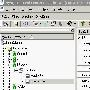# 用Perl的WriteExcel從數據庫生成電子數據表格文件

use DBI;

# connect to the local database

my \$dbh = DBI-connect('dbi:Oracle:','scott','tiger') die \$DBI::errstr;

my \$workbook = new Spreadsheet::WriteExcel "emp.xls";

my \$fmt = {

bottom=2,bg_color='silver'),

num_format='\$#,##0.00',align='right'),

num_format='\$#,##0.00',align='right',bold=1,

top=2),

};

print "Querying Employees\n";

my \$sth = \$dbh-prepare('select * from emp') die \$DBI::errstr;

my \$rc = \$sth-execute;

my \$r = 1;

\$r++;

while (my \$row = \$sth-fetchrow_hashref)

{

\$worksheet-write(\$r-1,0,\$row-{ENAME},\$fmt-{ENAME});

\$worksheet-write(\$r-1,1,\$row-{SAL},\$fmt-{MONEY});

\$worksheet-write(\$r-1,2,\$row-{COMM},\$fmt-{MONEY});

\$worksheet-write(\$r-1,3,"=B\$r+C\$r",\$fmt-{MONEY});

\$r++;

}

\$worksheet-write(\$r-1,0,'',\$fmt-{TOTAL});

\$worksheet-write(\$r-1,1,'=SUM(B2:B'.(\$r-1).')',\$fmt-{TOTAL});

\$worksheet-write(\$r-1,2,'=SUM(C2:C'.(\$r-1).')',\$fmt-{TOTAL});

\$worksheet-write(\$r-1,3,"=B\$r+C\$r",\$fmt-{TOTAL});

print "Processed " . (\$r-2) ." rows\n";

\$sth-finish;

許多商業最終用戶更喜歡使用目前流行的電子數據表格程序（如Microsoft Excel），而不是數據庫功能或應用程序。盡管Excel可以用ODBC及相關技術來提取儲存在Oracle數據庫當中的數據，但想讓用戶建立一個環境來直接...查看完整版>>用Perl的WriteExcel從數據庫生成電子數據表格文件

Hibernate技巧（1）：利用配置文件編寫程序生成數據庫
目前很多人使用Hibernate作爲持久層，假如我們已經寫了配置文件poweracl.hbm.XML，則不必再費勁寫SQL的DDL。除了利用工具SchemaEXPort之外，還可以編寫程序來自動初始化數據庫，並且生成SQL DDL。(1)Hibernate配...查看完整版>>Hibernate技巧（1）：利用配置文件編寫程序生成數據庫非凡說明：希望朋友們在引用尊重版權，因爲這是對像我這樣的朋友的最大尊重！況且這是我2006的處女貼,也希望對大家有點用，工資狂飙升！！1. 首先是預備開源包，到http://jdom.org.下載，當前最新版本是jdom-...查看完整版>>利用jdom實現讀取數據庫生成XML及讀取XML文件插入數據庫

Hibernate:利用配置文件生成數據庫